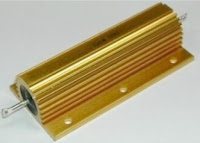# Resistors and Power Rating

Resistors find wide application in electrical and electronic circuits.  Resistors are used  in a wide range of applications such as to induce voltage drop, in filters, for energy dissipation and to limit current.While choosing a resistor it is necessary to ensure that the resistor has the proper wattage rating.  The wattage rating determines the amount of heat energy the resistors can absorb or dissipate.  When a resistor is selected, its wattage should also be checked for suitability to the application.  Selecting a resistors with the right ohmic value but the wrong wattage can cause serious damage to the resistor due to overheating.

When current flows through a resistor, heat is generated in accordance with Joule's Law.  H=I2 x R. The resistor should be designed to withstand the heat generated.  The heat generated in a resistor is proportional the current which flows through it.  The current, in turn, is proportional to the voltage across the resistor.

Power = V x I

Now I=V/R

Therefore Power = V2/R

The Power rating for the resistor can thus be calculated using the above formula.

High Power Resistors  with ratings of around 50 Watts are designed to be large in size (the large surface area dissipates heat).  The resistors are located away from other components to provide for ventilation.